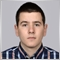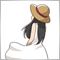# MQL5 custom indicator not calculating % correct3535

Hello,

I have a problem making my indicator calculating correct %, if it is less than 100% it returns 0% always. I printed the values, checked and did manual calculations, the percent should be ok, but not.

```     BuyBuffer[i] = (total_signalsB/totalindicators(0))*100;
SellBuffer[i] = (total_signalsS/totalindicators(0))*100;
Print("total s ",total_signalsS," total ",totalindicators(0)," % ",((total_signalsS/totalindicators(0))*100));
total_signalsB=0;
total_signalsS=0;
```

this code is a part of the main on calculate loop.

Example : the total signals B returns 1, the total_indicators(0) return 4 , 4 is the max amount of signals, therefore it should return 25% but returns 0.

What did I miss is the calculation ?390

```double total_signalsB  = 1;
double totalindicators = 4;

BuyBuffer[i] = (total_signalsB / totalindicators) * 100;  // return 25
```

In this case, it returns 25.

```int total_signalsB  = 1;
int totalindicators = 4;

BuyBuffer[i] = (total_signalsB / totalindicators) * 100;  // return 0```

But in this case, it returns 0.

Please check whether variables are double or int.3535

the variables are int, maybe thats the reason !3535